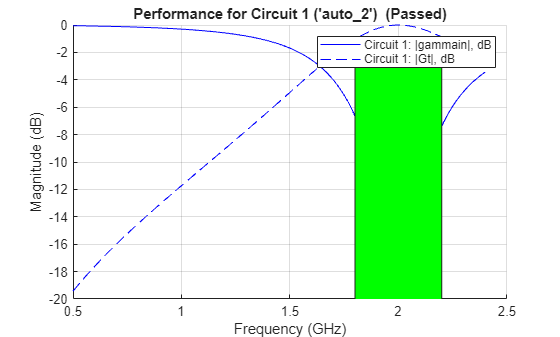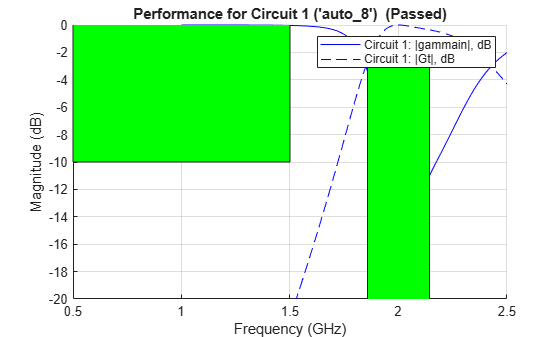# matchingnetwork

Create matching network for 1-port network and generate circuit object

## Description

Use the `matchingnetwork` object to create a matching network circuit for a 1-port network which match the impedance of given source to the impedance of given load at a specified center frequency. The `matchingnetwork` object stores the generated network as a `circuit` object in the Circuit property. The function `exportCircuits` could be also used to export the selected circuit(s) generated.

## Creation

### Syntax

``matchnet = matchingnetwork``
``matchnet = matchingnetwork(Name,Value)``

### Description

example

````matchnet = matchingnetwork` creates a matching network object with default property values.```

example

````matchnet = matchingnetwork(Name,Value)` sets properties using one or more name-value pairs. For example, `matchnet = matchingnetwork('SourceImpedance','60')` creates a matching network with a source impedance of 60 ohms.```

## Properties

expand all

Source impedance as seen at the terminals looking from the network into the source, specified as one of the following:

Example: `'SourceImpedance',60`

Example: `matchnet.SourceImpedance = 60`

Example: `'SourceImpedance','default.s1p'`

Data Types: `double` | `char` | `string` | `function_handle`

Load impedance as seen at the terminals looking from the matching network into the load, specified as one of the following:

Example: `'LoadImpedance',60`

Example: `matchnet.LoadImpedance = 60`

Data Types: `double` | `char` | `string` | `function_handle`

Frequency to calculate the impedance match between the source and the load, specified as a real positive scalar in hertz

Example: `'CenterFrequency',1e9`

Example: `matchnet.CenterFrequency = 1e9`

Data Types: `double`

Desired bandwidth (transducer gain >= minus 3 dB over this bandwidth centered on `CenterFrequency`), specified as a real positive scalar in hertz.

Example: `'BandWidth',100e6`

Example: `matchnet.BandWidth = 100e6`

Data Types: `double`

Desired loaded quality factor, specified as a real positive scalar. Setting `LoadedQ` updates the bandwidth. If you specify `CenterFrequency`, `LoadedQ` is recalculated from `CenterFrequency` and `BandWidth`.

Example: `'LoadedQ',2`

Example: `matchnet.LoadedQ = 2`

Data Types: `double`

Note

The addition of a third element introduces an added degree of freedom allowing you to control the `LoadedQ` property. Hence, the `Bandwidth` and the `LoadedQ` are hidden when there are two components. For more information please see, .

Number of components or type of topology for the matching network design, specified as `2` or `3` for the number of components and `'Pi'`, `'Tee'`, or, `'L'` for the type of topology.

Example: `'Components','Pi'`

Example: `matchnet.Components = 'Pi'`

Data Types: `double` | `char` | `string`

An array of circuit objects containing possible matching network designs for the given set of parameters.

Note

## Object Functions

 `addEvaluationParameter` Adds performance goal for sort, pass, or fail matching network design `circuitDescriptions` Tables describing each created matching network's topology and performance `getEvaluationParameters` Table of evaluation parameters currently used to rank and pass or fail matching network designs `clearEvaluationParameter` Delete one or more performance goals `exportCircuits` Select and export generated matching networks as circuit objects from an existing matching network object `rfplot` Plot cumulative RF budget result versus cascade input frequency `smithplot` Plot measurement data on Smith chart `sparameters` S-parameter object

## Examples

collapse all

Create a default matching network using the object, `matchingnetwork`.

`matchnet = matchingnetwork`
```matchnet = matchingnetwork with properties: SourceImpedance: 50 Ohms LoadImpedance: 50 Ohms CenterFrequency: 1 GHz Components: 2 Circuit: [1x2 circuit] ```

Create a matching network with source impedance, 100 ohms, load impedance, 75 ohms, center frequency, 2 GHz, desired loaded quality factor, 5, and the number of components, 3.

```mnobj = matchingnetwork('SourceImpedance',100,'LoadImpedance',... 75,'CenterFrequency',2e9,'LoadedQ',5,'Components',3)```
```mnobj = matchingnetwork with properties: SourceImpedance: 100 Ohms LoadImpedance: 75 Ohms CenterFrequency: 2 GHz Bandwidth: 400 MHz Components: 3 LoadedQ: 5 Circuit: [1x8 circuit] ```

Display the list of matching network circuits generated and their corresponding performance

`[circuit_list, performance] = circuitDescriptions(mnobj)`
```circuit_list=8×7 table circuitName component1Type component1Value component2Type component2Value component3Type component3Value ___________ ______________ _______________ ______________ _______________ ______________ _______________ Circuit 1 "auto_2" "Shunt C" 3.9789e-12 "Series L" 2.1389e-10 "Shunt L" 1.3876e-09 Circuit 2 "auto_7" "Series C" 1.8501e-13 "Shunt C" 2.8519e-14 "Series L" 2.9842e-08 Circuit 3 "auto_3" "Shunt L" 1.5915e-09 "Series C" 2.9607e-11 "Shunt C" 4.5637e-12 Circuit 4 "auto_6" "Series L" 3.4228e-08 "Shunt L" 2.2205e-07 "Series C" 2.1221e-13 Circuit 5 "auto_1" "Shunt C" 3.9789e-12 "Series L" 2.8468e-09 "Shunt C" 4.5637e-12 Circuit 6 "auto_5" "Series L" 3.4228e-08 "Shunt C" 3.7957e-13 "Series L" 2.9842e-08 Circuit 7 "auto_4" "Shunt L" 1.5915e-09 "Series C" 2.2245e-12 "Shunt L" 1.3876e-09 Circuit 8 "auto_8" "Series C" 1.8501e-13 "Shunt L" 1.6684e-08 "Series C" 2.1221e-13 ```
```performance=8×4 table circuitName evaluationPassed testsFailed performanceScore ___________ ________________ ____________ ________________ Circuit 1 "auto_2" {["Yes"]} {0x0 double} {[ 1.9447]} Circuit 2 "auto_7" {["Yes"]} {0x0 double} {[ 1.9447]} Circuit 3 "auto_3" {["Yes"]} {0x0 double} {[ 1.9443]} Circuit 4 "auto_6" {["Yes"]} {0x0 double} {[ 1.9443]} Circuit 5 "auto_1" {["No" ]} {[ 1]} {[-0.1254]} Circuit 6 "auto_5" {["No" ]} {[ 1]} {[-0.1254]} Circuit 7 "auto_4" {["No" ]} {[ 1]} {[-0.6947]} Circuit 8 "auto_8" {["No" ]} {[ 1]} {[-0.6947]} ```

Plot the frequency response of the best circuit (Circuit #1) between 0.5 GHz and 2.5 GHz.

```frequencies = linspace(0.5e9,2.4e9); CircuitIndex = 1; % Best circuit is sorted to the top rfplot(mnobj,frequencies,CircuitIndex)```Plot impedance transformation for the best matching network generated (Circuit#1). For more information, see `smithplot`.

`smithplot(mnobj)`To export a selected matching network circuit, for example, Circuit #5:

```CircuitIndex = 5; mn_circuit = mnobj.Circuit(CircuitIndex)```
```mn_circuit = circuit: Circuit element ElementNames: {'C' 'L' 'C_1'} Elements: [1x3 rf.internal.circuit.RLC] Nodes: [1 2 3] Name: 'unnamed' NumPorts: 2 Terminals: {'p1+' 'p2+' 'p1-' 'p2-'} ```

Alternatively, use exportCircuits(m,CircuitIndex).

Show the default evaluation parameters used by the matching network.

`ep = getEvaluationParameters(mnobj)`
```ep=1×6 table Parameter Comparison Goal Band Weight Source _________ __________ ______ ____________ ______ _____________ {'Gt'} {'>'} {[-3]} {1x2 double} {} {'Automatic'} ```

Add a new evaluation parameter and plot the frequency response of Circuit #1.

`mnobj = mnobj.addEvaluationParameter('gammain','>',-2,[0.5e9 1.5e9],1)`
```mnobj = matchingnetwork with properties: SourceImpedance: 100 Ohms LoadImpedance: 75 Ohms CenterFrequency: 2 GHz Bandwidth: 400 MHz Components: 3 LoadedQ: 5 Circuit: [1x8 circuit] ```
`rfplot(mnobj,frequencies,1)`Create a dipole antenna and create the S-parameters of the antenna. This example requires Antenna Toolbox.

```d = dipole('Length', 0.103, 'Width',0.0022); freq = linspace(0.5e9,2.5e9,1001); sd = sparameters(d, freq);```

Alternatively, load S-Parameters from the MAT file

`% load('sparams_dipole.mat')`

Create a matching network from the S-parameters.

```n = matchingnetwork('LoadImpedance',sd,'Components',3,... 'LoadedQ',7,'CenterFrequency',2e9);```

Get the evaluation parameters of the network.

`t = getEvaluationParameters(n)`
```t=1×6 table Parameter Comparison Goal Band Weight Source _________ __________ ______ ____________ ______ _____________ {'Gt'} {'>'} {[-3]} {1x2 double} {} {'Automatic'} ```

Plot the reflection coefficient and transducer gain of the matching network circuit 1 , at a frequency range of 1 GHz to 2.5 GHz.

`rfplot(n, (1e9:0.001e9:2.5e9),1);`Add a new evaluation parameter to compare the transducer gain to have a cut-off of less than -10 dB. Use a frequency range of 0.5 GHz to 1.5 GHz. Plot the comparisons.

```n = addEvaluationParameter(n, 'Gt', '<', -10, [0.5e9 1.5e9], 1); t = getEvaluationParameters(n)```
```t=2×6 table Parameter Comparison Goal Band Weight Source _________ __________ _______ ____________ ______ __________________ {'Gt'} {'>'} {[ -3]} {1x2 double} {} {'Automatic' } {'Gt'} {'<'} {[-10]} {1x2 double} {} {'User-specified'} ```
`rfplot(n, (1e9:0.001e9:2.5e9),1);`Clear evaluation parameters.

```n = clearEvaluationParameter(n,1); t = getEvaluationParameters(n)```
```t=1×6 table Parameter Comparison Goal Band Weight Source _________ __________ _______ ____________ ______ __________________ {'Gt'} {'<'} {[-10]} {1x2 double} {} {'User-specified'} ```

This example shows how to calculate the S-Parameters for a newly created matching network for the auto-generated circuit #2 with a reference impedance of 100 Ohm.

```n = matchingnetwork('LoadImpedance',100,'Components',3); freq = linspace(n.CenterFrequency-n.Bandwidth/2,n.CenterFrequency+n.Bandwidth/2); RefZ0 = 100; ckt_no = 2; s = sparameters(n,freq,RefZ0,ckt_no)```
```s = sparameters: S-parameters object NumPorts: 2 Frequencies: [100x1 double] Parameters: [2x2x100 double] Impedance: 100 rfparam(obj,i,j) returns S-parameter Sij ```

 Ludwig, Reinhold, and Gene Bogdanov. RF Circuit Design: Theory and Applications. Prentice-Hall, 2009.

 Bowick, Chris, et al. RF Circuit Design. 2nd ed, 2008.